Main: AnIdentityForKernelRidgeRegression

This paper describes an approach to prove theoretical guarantees on online kernelized Ridge Regression in the form of equality. It approaches the results described in Zhdanov and Vovk, 2009, from the probabilistic perspective.

The method of kernel Ridge Regression with a kernel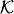and a real regularisation parameter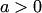suggests the function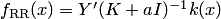, where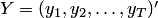is the column vector of outcomes,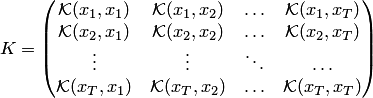is the kernel matrix and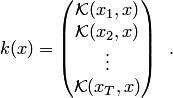Theorem. Take a kernelon a domain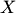and a parameter. Let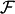be the RKHS for the kernel. For a sample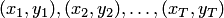let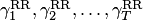be the predictions output by ridge regression with the kerneland the parameter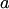in the on-line mode. Then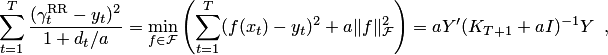where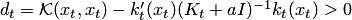.

The idea of the proof is very elegant: the density of the joint distribution of the variables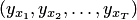at the point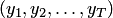is calculated in three different ways: by decomposing the density into a chain of conditional densities, marginalisation, and, finally, direct calculation. Each method will give us a different expression corresponding to a term in the identity. Since all the three terms express the same density, they must be equal.

The following corollary is also proven on the cumulative square loss of the clipped kernel Ridge Regression.

Corollary. Take a kernelon a domainand a parameter. Letbe the RKHS for the kernel. For a samplesuch that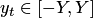for all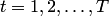, let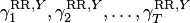be the predictions output by clipped ridge regression with the kerneland the parameterin the on-line mode. Then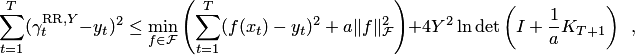where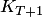is as above.

• Fedor Zhdanov and Vladimir Vovk. Competing with Gaussian linear experts. Technical report, arXiv:0910.4683 [cs.LG], arXiv.org e-Print archive, 2009.
• Fedor Zhdanov and Yuri Kalnishkan. An identity for kernel ridge regression. In Proceedings of the 21st International Conference on Algorithmic Learning Theory, 2010.